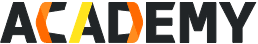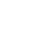#New User FREE Learning Path

Step 1: Claim the New User Benefit

Step 2: Redeem FREE Clouders to learn

Step 3: Get a FREE ACA Certification Exam

Operate and Manage a Cloud Server

Free

• Certification
• Machine Learning Algorithm Primer Series 3- Logistic Regression Model
Machine Learning Algorithm Primer Series 3- Logistic Regression Model• Certification
• Machine Learning Algorithm Primer Series 3- Logistic Regression Model
Machine Learning Algorithm Primer Series 3- Logistic Regression ModelClouder at a Glance
• Video Course Duration
45 Minutes
• Available Languages
English
• Course Type
Online
USD 10.00
Introduction
This course is the 3rd class of the Alibaba Cloud Machine Learning Algorithm QuickStart series, It mainly introduces the basic concept and theory on Logistic Regression Model, as well as the data preprocessing technique in Logistic Regression Model, implements Logistic Regression Model with PAI to reslove business problem, prepar for the knowledge associate with subsequent machine learning courses.
Recommended For
Machine Learning beginners, cloud computing beginners
How to get Certified
Exam Overview
• Certification:
Apsara Clouder - Big Data: Machine Learning Algorithm Primer Series 3- Logistic Regression Model
• Exam Type:
Online
• Available Languages:
English
• Exam Duration:
30 Minutes
• No. of Exam Attempts:
2 Times
Courses
Machine Learning Algorithm Primer Series 3- Logistic Regression Model
How to apply logistic regression model to solve prediction and classification problems with PAI.
Show Details
•Course objective
•Understand the basic concepts of Logistic Regression Model
•Familiar with Logistic Regression Model theory
•Master the data preprocessing technique in Logistic Regression Model
•Hands-on: Applying Logistic Regression Model with PAI
Recommended Certifications